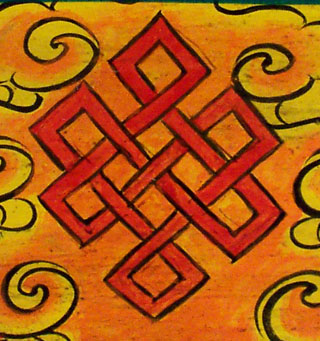## WHAT: Riemann Surfaces and Algebraic Curves## Goal of this class is to explore the interconnections of the analytic theory of Riemann Surfaces and the algebro-geometric theory of Algebraic Curves. We may take a detour into combinatorics, representation theory of the symmetric group, and maybe even a little bit of physics, depending on how things evolve.

HOMEWORK

[SEP. 20]  1. Prove that a bi-homogeneous polynomial F in X_0, X_1, Y_0,Y_1 defines a Riemann Surface  C in P^1xP^1 if and only if there is no point P in C where F, one of the                          partials with respect to the X's and one of the partials with respect to the Y's vanish simultaneously.
2. Prove that a (projective) plane conic is isomorphic to P^1.
3. Prove (carefully) that functions P^1 -> P^1 are precisely  rational  functions of one affine local coordinate, or, alternatively, homogeneous rational functions of degree zero                     in the homogeneous coordinates.
[OCT. 23]  1. Consider a line bundle O(k) on P^1. Write down the transition function for the trivialization of O(k) over the standard covering of P^1 consisting of the two open sets     missing 0 and infinity.  When you restrict a section to either trivialization you get a function. Show that the transition between these local functions has opposite exponent than the one on the charts. (explicitly, a section which is constant on one chart will become the polynomial z^k on the other). Think of a philosophical explanation for this phenomenon.
2. Prove that the cotangent bundle of P^1 is isomorphic to O(-2), and the tangent bundle of P^1 is isomorphic to O(2).
3. The tautological bundle on P^1 is the subbundle of P^1x C^2 such that the fiber of each point of P^1 is exactly the line parameterized by the point. Write down equations for this condition. Show that this line bundle is isomorphic to O(-1).

BOOKS:

There are three books I am thinking of using (parts of) for this class:

1·        R. Miranda. Algebraic Curves and Riemann Surfaces.

2·        R. Cavalieri, E. Miles. Riemann Surfaces and Algebraic Curves: a first course in Hurwitz Theory.

3·      E. Arbarello, M. Cornalba, P. Griffiths, J. Harris. Algebraic Curves.